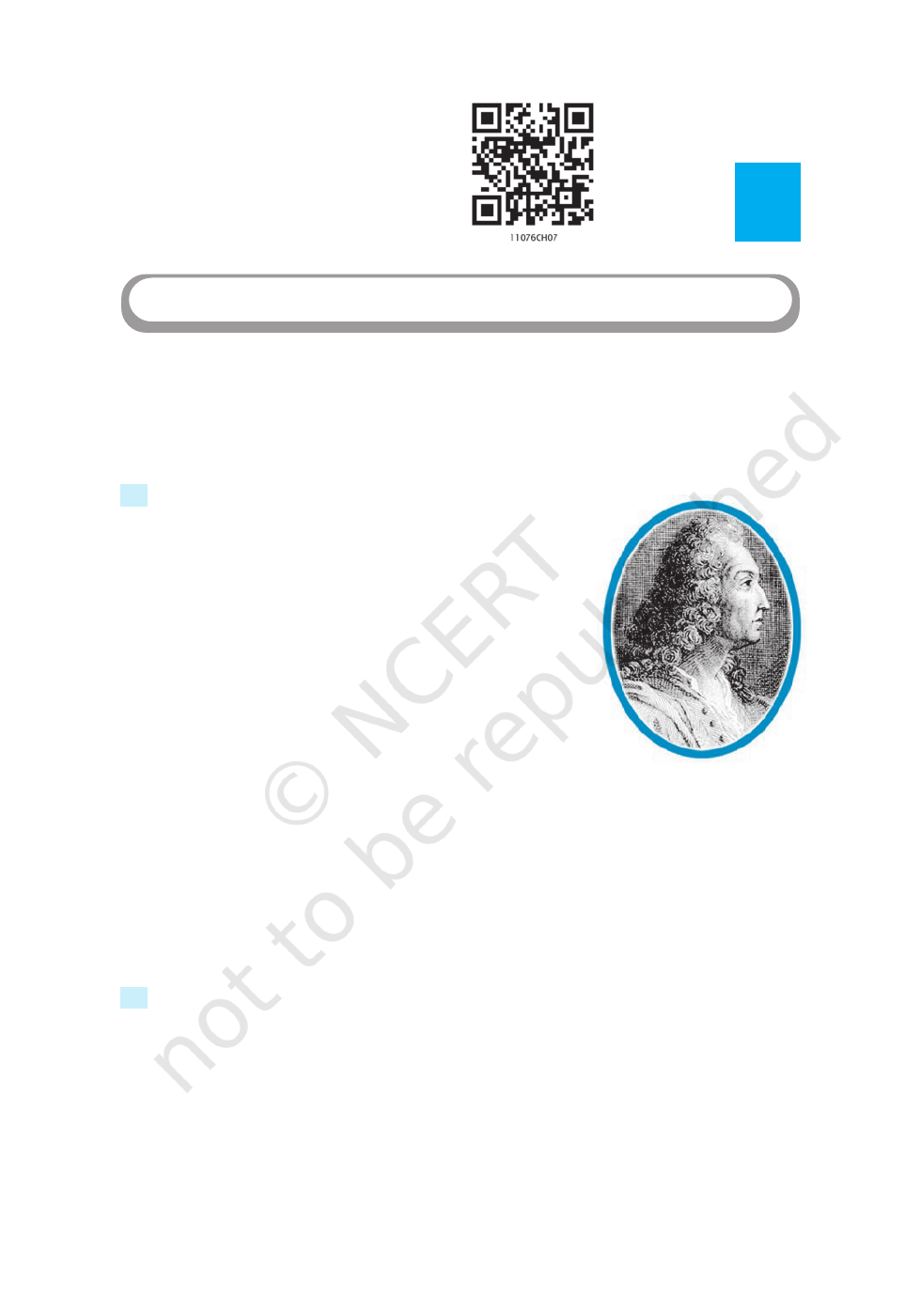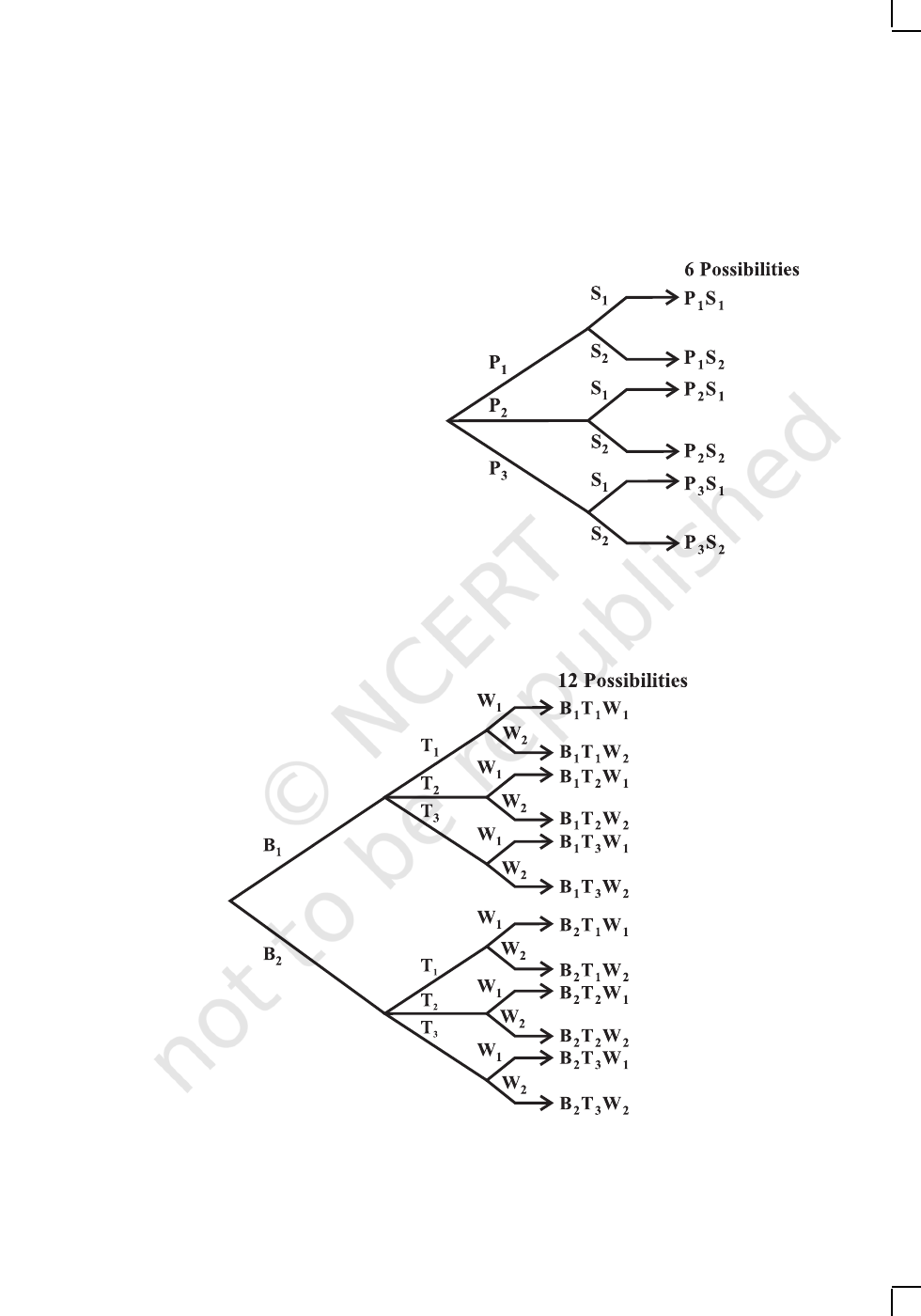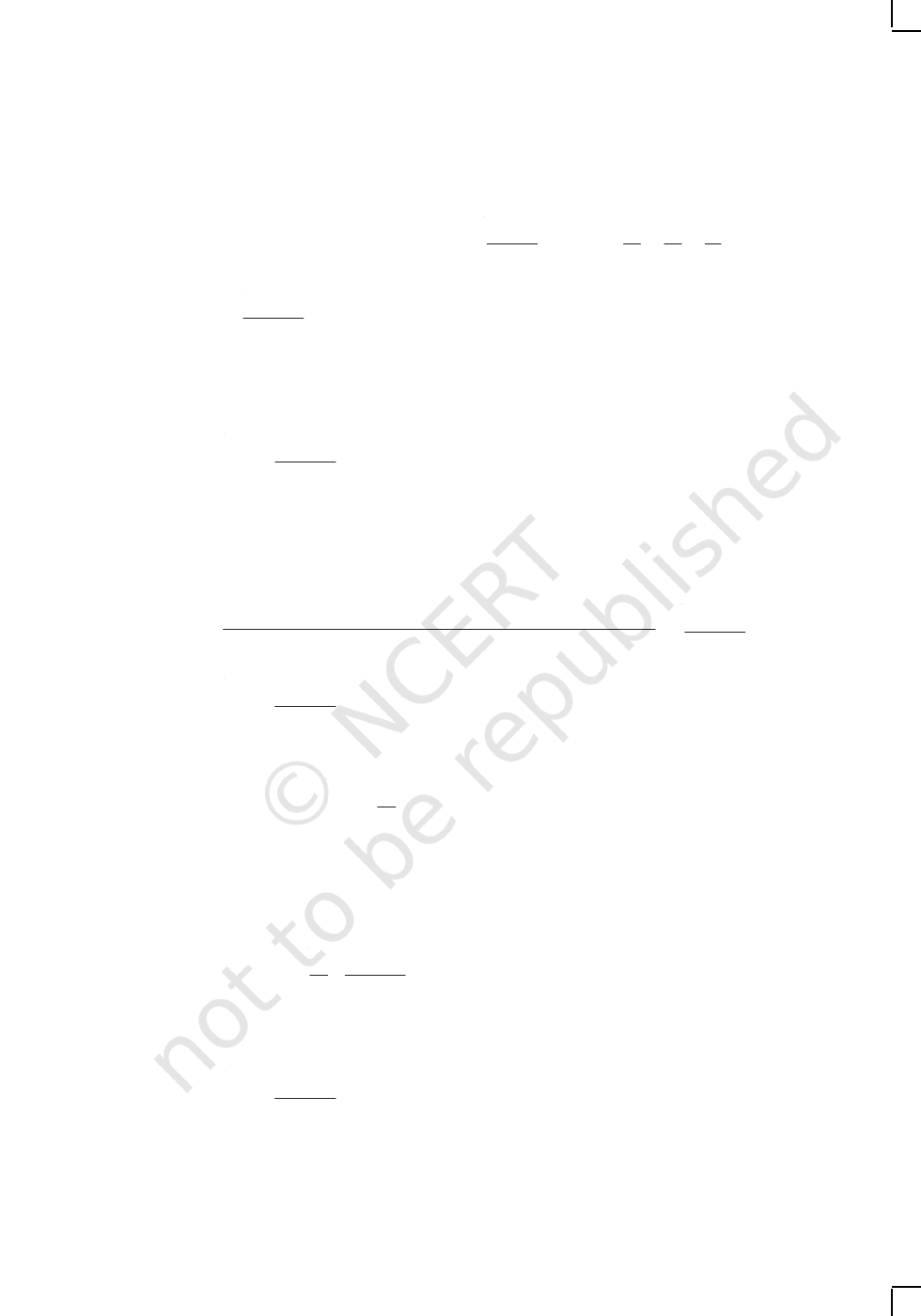Jacob Bernoulli
(1654-1705)
vEvery body of discovery is mathematical in form because there is no
other guidance we can have – DARWINv
7.1 Introduction
Suppose you have a suitcase with a number lock. The number
lock has 4 wheels each labelled with 10 digits from 0 to 9.
The lock can be opened if 4 specific digits are arranged in a
particular sequence with no repetition. Some how, you have
forgotten this specific sequence of digits. You remember only
the first digit which is 7. In order to open the lock, how
many sequences of 3-digits you may have to check with? To
answer this question, you may, immediately, start listing all
possible arrangements of 9 remaining digits taken 3 at a
time. But, this method will be tedious, because the number
of possible sequences may be large. Here, in this Chapter,
we shall learn some basic counting techniques which will enable us to answer this
question without actually listing 3-digit arrangements. In fact, these techniques will be
useful in determining the number of different ways of arranging and selecting objects
without actually listing them. As a first step, we shall examine a principle which is most
fundamental to the learning of these techniques.
7.2 Fundamental Principle of Counting
Let us consider the following problem. Mohan has 3 pants and 2 shirts. How many
different pairs of a pant and a shirt, can he dress up with? There are 3 ways in which
a pant can be chosen, because there are 3 pants available. Similarly, a shirt can be
chosen in 2 ways. For every choice of a pant, there are 2 choices of a shirt. Therefore,
there are 3 × 2 = 6 pairs of a pant and a shirt.
7
Chapter
PERMUTATIONS AND COMBINATIONS
2020-21PERMUTATIONS AND COMBINATIONS 135
Let us name the three pants as P
1
, P
2
, P
3
and the two shirts as S
1
, S
2
. Then,
these six possibilities can be illustrated in the Fig. 7.1.
Let us consider another problem
of the same type.
Sabnam has 2 school bags, 3 tiffin boxes
and 2 water bottles. In how many ways
can she carry these items (choosing one
each).
A school bag can be chosen in 2
different ways. After a school bag is
chosen, a tiffin box can be chosen in 3
different ways. Hence, there are
2 × 3 = 6 pairs of school bag and a tiffin
box. For each of these pairs a water
bottle can be chosen in 2 different ways.
Hence, there are 6 × 2 = 12 different ways in which, Sabnam can carry these items to
school. If we name the 2 school bags as B
1
, B
2
, the three tiffin boxes as T
1
, T
2
, T
3
and
the two water bottles as W
1
, W
2
, these possibilities can be illustrated in the Fig. 7.2.
Fig 7.1
Fig 7.2
2020-21136 MATHEMATICS
In fact, the problems of the above types are solved by applying the following
principle known as the fundamental principle of counting, or, simply, the multiplication
principle, which states that
“If an event can occur in m different ways, following which another event
can occur in n different ways, then the total number of occurrence of the events
in the given order is m×n.”
The above principle can be generalised for any finite number of events. For
example, for 3 events, the principle is as follows:
‘If an event can occur in m different ways, following which another event can
occur in n different ways, following which a third event can occur in p different ways,
then the total number of occurrence to ‘the events in the given order is m × n × p.”
In the first problem, the required number of ways of wearing a pant and a shirt
was the number of different ways of the occurence of the following events in succession:
(i) the event of choosing a pant
(ii) the event of choosing a shirt.
In the second problem, the required number of ways was the number of different
ways of the occurence of the following events in succession:
(i) the event of choosing a school bag
(ii) the event of choosing a tiffin box
(iii) the event of choosing a water bottle.
Here, in both the cases, the events in each problem could occur in various possible
orders. But, we have to choose any one of the possible orders and count the number of
different ways of the occurence of the events in this chosen order.
Example 1
Find the number of 4 letter words, with or without meaning, which can be
formed out of the letters of the word ROSE, where the repetition of the letters is not
allowed.
Solution
There are as many words as there are ways of filling in 4 vacant places
by the 4 letters, keeping in mind that the repetition is not allowed. The
first place can be filled in 4 different ways by anyone of the 4 letters R,O,S,E. Following
which, the second place can be filled in by anyone of the remaining 3 letters in 3
different ways, following which the third place can be filled in 2 different ways; following
which, the fourth place can be filled in 1 way. Thus, the number of ways in which the
4 places can be filled, by the multiplication principle, is 4 × 3 × 2 × 1 = 24. Hence, the
required number of words is 24.
2020-21PERMUTATIONS AND COMBINATIONS 137
A
Note If the repetition of the letters was allowed, how many words can be formed?
One can easily understand that each of the 4 vacant places can be filled in succession
in 4 different ways. Hence, the required number of words = 4 × 4 × 4 × 4 = 256.
Example 2
Given 4 flags of different colours, how many different signals can be
generated, if a signal requires the use of 2 flags one below the other?
Solution
There will be as many signals as there are ways of filling in 2 vacant places
in succession by the 4 flags of different colours. The upper vacant place can
be filled in 4 different ways by anyone of the 4 flags; following which, the lower vacant
place can be filled in 3 different ways by anyone of the remaining 3 different flags.
Hence, by the multiplication principle, the required number of signals = 4 × 3 = 12.
Example 3 How many 2 digit even numbers can be formed from the digits
1, 2, 3, 4, 5 if the digits can be repeated?
Solution There will be as many ways as there are ways of filling 2 vacant places
in succession by the five given digits. Here, in this case, we start filling in unit’s
place, because the options for this place are 2 and 4 only and this can be done in 2
ways; following which the ten’s place can be filled by any of the 5 digits in 5 different
ways as the digits can be repeated. Therefore, by the multiplication principle, the required
number of two digits even numbers is 2 × 5, i.e., 10.
Example 4 Find the number of different signals that can be generated by arranging at
least 2 flags in order (one below the other) on a vertical staff, if five different flags are
available.
Solution A signal can consist of either 2 flags, 3 flags, 4 flags or 5 flags. Now, let us
count the possible number of signals consisting of 2 flags, 3 flags, 4 flags and 5 flags
separately and then add the respective numbers.
There will be as many 2 flag signals as there are ways of filling in 2 vacant places
in succession by the 5 flags available. By Multiplication rule, the number of
ways is 5 × 4 = 20.
Similarly, there will be as many 3 flag signals as there are ways of filling in 3
vacant places in succession by the 5 flags.
2020-21138 MATHEMATICS
The number of ways is 5 × 4 × 3 = 60.
Continuing the same way, we find that
The number of 4 flag signals = 5 × 4 × 3 × 2 = 120
and the number of 5 flag signals = 5 × 4 × 3 × 2 × 1 = 120
Therefore, the required no of signals = 20 + 60 + 120 + 120 = 320.
EXERCISE 7.1
1. How many 3-digit numbers can be formed from the digits 1, 2, 3, 4 and 5
assuming that
(i) repetition of the digits is allowed?
(ii) repetition of the digits is not allowed?
2. How many 3-digit even numbers can be formed from the digits 1, 2, 3, 4, 5, 6 if the
digits can be repeated?
3. How many 4-letter code can be formed using the first 10 letters of the English
alphabet, if no letter can be repeated?
4. How many 5-digit telephone numbers can be constructed using the digits 0 to 9 if
each number starts with 67 and no digit appears more than once?
5. A coin is tossed 3 times and the outcomes are recorded. How many possible
outcomes are there?
6. Given 5 flags of different colours, how many different signals can be generated if
each signal requires the use of 2 flags, one below the other?
7.3 Permutations
In
Example 1 of the previous Section, we are actually counting the different possible
arrangements of the letters such as ROSE, REOS, ..., etc. Here, in this list, each
arrangement is different from other. In other words, the order of writing the letters is
important. Each arrangement is called a permutation of 4 different letters taken all
at a time. Now, if we have to determine the number of 3-letter words, with or without
meaning, which can be formed out of the letters of the word NUMBER, where the
repetition of the letters is not allowed, we need to count the arrangements NUM,
NMU, MUN, NUB, ..., etc. Here, we are counting the permutations of 6 different
letters taken 3 at a time. The required number of words = 6 × 5 × 4 = 120 (by using
multiplication principle).
If the repetition of the letters was allowed, the required number of words would
be 6 × 6 × 6 = 216.
2020-21PERMUTATIONS AND COMBINATIONS 139
Definition 1
A permutation is an arrangement in a definite order of a number of
objects taken some or all at a time.
In the following sub-section, we shall obtain the formula needed to answer these
questions immediately.
7.3.1 Permutations when all the objects are distinct
Theorem 1
The number of permutations of n different objects taken r at a time,
where 0 < r n and the objects do not repeat is n ( n – 1) ( n – 2). . .(
nr + 1),
which is denoted by
n
P
r
.
Proof There will be as many permutations as there are ways of filling in r vacant
places
. . . by
r vacant places
the n objects. The first place can be filled in n ways; following which, the second place
can be filled in (n – 1) ways, following which the third place can be filled in (n – 2)
ways,..., the rth place can be filled in (n – (r – 1)) ways. Therefore, the number of
ways of filling in r vacant places in succession is n(n – 1) (n – 2) . . . (n – (r – 1)) or
n ( n – 1) (n – 2) ... (n r + 1)
This expression for
n
P
r
is cumbersome and we need a notation which will help to
reduce the size of this expression. The symbol n! (read as factorial n or n factorial )
comes to our rescue. In the following text we will learn what actually n! means.
7.3.2 Factorial notation The notation n! represents the product of first n natural
numbers, i.e., the product 1 × 2 × 3 × . . . × (n – 1) × n is denoted as n!. We read this
symbol as ‘n factorial’. Thus, 1 × 2 × 3 × 4 . . . × (n – 1) × n = n !
1 = 1 !
1 × 2 = 2 !
1× 2 × 3 = 3 !
1 × 2 × 3 × 4 = 4 ! and so on.
We define 0 ! = 1
We can write 5 ! = 5 × 4 ! = 5 × 4 × 3 ! = 5 × 4 × 3 × 2 !
= 5 × 4 × 3 × 2 × 1!
Clearly, for a natural number n
n ! = n (n –
1) !
= n (n – 1) (n – 2) ! [provided (n 2)]
= n (n – 1) (n – 2) (n – 3) ! [provided (n 3)]
and so on.
2020-21140 MATHEMATICS
Example 5 Evaluate (i) 5 ! (ii) 7 ! (iii) 7 ! – 5!
Solution (i) 5 ! = 1 × 2 × 3 × 4 × 5 = 120
(ii) 7 ! = 1 × 2 × 3 × 4 × 5 × 6 ×7 = 5040
and (iii) 7 ! – 5! = 5040 – 120 = 4920.
Example 6 Compute (i)
7!
5!
(ii)
( )
12!
10! (2!)
Solution (i) We have
7!
5!
=
7 6 5!
× ×
= 7 × 6 = 42
and (ii)
( ) ( )
12!
10! 2!
=
(
)
( ) ( )
12 11 10!
10! 2
× ×
×
= 6 × 11 = 66.
Example 7 Evaluate
( )
!
! !
n
r n r
, when n = 5, r = 2.
Solution We have to evaluate
( )
5!
2! 5 2 !
(since n = 5, r = 2)
We have
( )
5!
2 ! 5 2 !
=
5! 5 4
10
2! 3! 2
×
= =
×
.
Example 8 If
1 1
8! 9! 10!
x
+ =
, find x.
Solution We have
1 1
8! 9 8! 10 9 8!
x
+ =
× × ×
Therefore
1
1
9 10 9
x
+ =
×
or
10
9 10 9
x
=
×
So x = 100.
EXERCISE 7.2
1. Evaluate
(i) 8 ! (ii) 4 ! – 3 !
2020-21PERMUTATIONS AND COMBINATIONS 141
2. Is 3 ! + 4 ! = 7 ! ? 3. Compute
8!
6! 2!
×
4. If
1 1
6! 7! 8!
x
+ =
, find x
5. Evaluate
( )
!
!
n
n r
, when
(i) n = 6, r = 2 (ii) n = 9, r = 5.
7.3.3 Derivation of the formula for
n
P
r
( )
!
P
!
n
r
n
n r
=
, 0 r n
Let us now go back to the stage where we had determined the following formula:
n
P
r
= n (n – 1) (n – 2) . . . (nr + 1)
Multiplying numerator and denomirator by (nr) (nr – 1) . . . 3 × 2 × 1, we get
(
)
(
)
(
)
(
)
(
)
( )( )
1 2 1 1 3 2 1
P
1 3 2 1
n
r
n n n ... n r n r n r ...
n r n r ...
+ × ×
=
× ×
=
( )
!
!
n
n r
,
Thus
( )
!
P
!
n
r
n
n r
=
, where 0 < r
n
This is a much more convenient expression for
n
P
r
than the previous one.
In particular, when r = n,
!
P !
0!
n
n
n
n
= =
Counting permutations is merely counting the number of ways in which some or
all objects at a time are rearranged. Arranging no object at all is the same as leaving
behind all the objects and we know that there is only one way of doing so. Thus, we
can have
n
P
0
= 1 =
! !
! ( 0)!
=
n n
n n
... (1)
Therefore, the formula (1) is applicable for r = 0 also.
Thus
( )
!
P 0
!
n
r
n
, r n
n r
=
.
2020-21